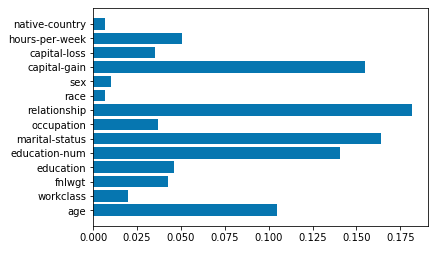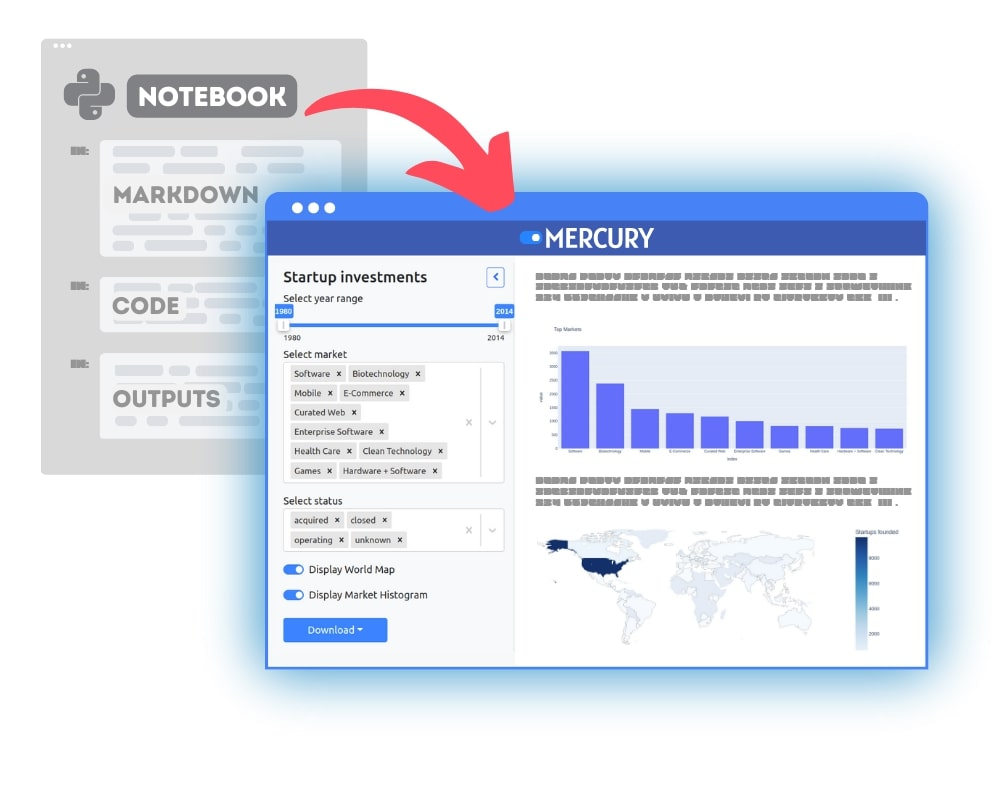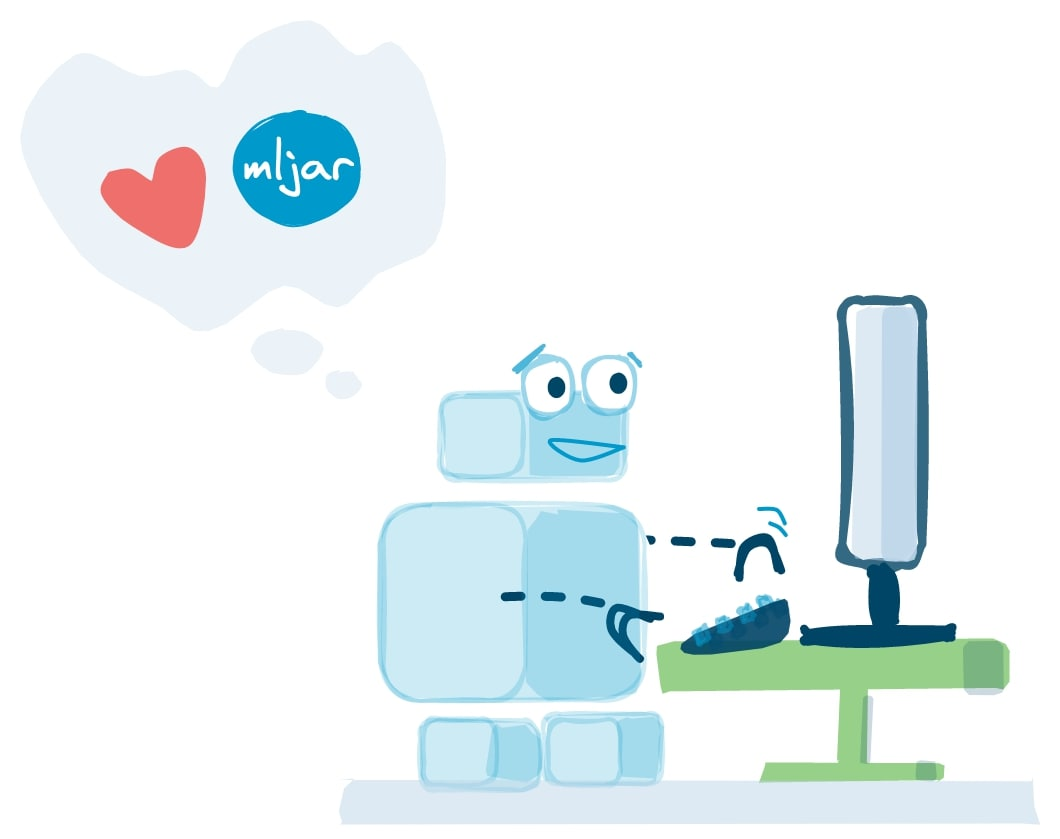Random Forest versus AutoML you say. Hmmm…, it’s obvious that the performance of AutoML will be better. You will check many models and then ensemble them. This is true, but I would like to show you other advantages of AutoML, that will help you deal with dirty, real-life data.

1. I will show you how to prepare the data and train Random Forest model on Adult dataset with python and scikit-learn.
2. On the same dataset, I will show you how to train Random Forest with AutoML mljar-supervised, which is an open source package developed by me :) You will see how AutoML can make your life easier when dealing with real-life, dirty data.

The link to the code in Jupyter Notebook is here.

## Random Forest in python and sklearn

Let’s load python packages that we will use.

``````# packages for data manipulation
import numpy as np
import pandas as pd
# packages to split data and compute logloss
import sklearn.model_selection
from sklearn.metrics import log_loss
# packages to train models
from sklearn.ensemble import RandomForestClassifier
from supervised.automl import AutoML
``````

As you see, loading `AutoML` package is as simple as importing `sklearn` package. Let’s load data, and split it into train and test subsets.

``````# load dataset
skipinitialspace=True)
x_cols = [c for c in df.columns if c != 'income']
# set input matrix and target column
X = df[x_cols]
y = df['income']
# split data into training and testing
seed = 1234
X_train, X_test, y_train, y_test = sklearn.model_selection.train_test_split(X, y,
test_size = 0.3, random_state=seed)
``````

As you see I use 30% of data for testing. Data is loaded from my github repository with a list of datasets which are good for starting the machine learning journey. Let’s take a preview of the data.OK, data is loaded so, let’s train machine learning model!

``````rf = RandomForestClassifier(n_estimators = 1000)
rf = rf.fit(X_train, y_train)
``````

As you see, training Random Forest with sklearn is simple (and as you will see further, training is simple, but preparing the data is not). In the first line, we initialize the model and in the second line, we train it. But wait, let’s execute the code. Bum! Errors!

``````---------------------------------------------------------------------------
ValueError                                Traceback (most recent call last)
1 rf = RandomForestClassifier(n_estimators = 1000)
----> 2 rf = rf.fit(X_train, y_train)

~/sandbox/rf/automl-rf/venv/lib/python3.6/site-packages/sklearn/ensemble/forest.py in
fit(self, X, y, sample_weight)
248
249         # Validate or convert input data
--> 250         X = check_array(X, accept_sparse="csc", dtype=DTYPE)
251         y = check_array(y, accept_sparse='csc', ensure_2d=False, dtype=None)
252         if sample_weight is not None:

~/sandbox/rf/automl-rf/venv/lib/python3.6/site-packages/sklearn/utils/validation.py in
check_array(array, accept_sparse, accept_large_sparse, dtype, order, copy, force_all_finite,
ensure_2d, allow_nd, ensure_min_samples, ensure_min_features, warn_on_dtype, estimator)
525             try:
526                 warnings.simplefilter('error', ComplexWarning)
--> 527                 array = np.asarray(array, dtype=dtype, order=order)
528             except ComplexWarning:
529                 raise ValueError("Complex data not supported\n"

~/sandbox/rf/automl-rf/venv/lib/python3.6/site-packages/numpy/core/numeric.py in
asarray(a, dtype, order)
536
--> 538     return array(a, dtype, copy=False, order=order)
539
540

ValueError: could not convert string to float: 'Private'
``````

We have `ValueError`, because there is ‘Private’ value in the `workclass` column. This column has categorical values. Hmmm …, this is strange, the Random Forest is an ensemble of decision trees, and decision trees should work with categorical values … Quick googling, and you got confirmation that scikit-learn Random Forest doesn’t work with categorical values and that somebody is working on this in sklearn (stackoverflow link). We need to convert our categorical columns into numerical values. The common option here is one-hot encoding or converting into integers. Let’s convert categorical values to integers.

``````from sklearn.preprocessing import LabelEncoder

for column in ['workclass', 'education', 'marital-status', 'occupation',
'relationship', 'race', 'sex','native-country']:
categorical_convert = LabelEncoder()
X_train[column] = categorical_convert.fit_transform(X_train[column])
X_test[column] = categorical_convert.transform(X_test[column])
``````

Execute the code, and … error again!

``````---------------------------------------------------------------------------
TypeError                                 Traceback (most recent call last)
3 for column in ['workclass', 'education', 'marital-status', 'occupation',
'relationship', 'race', 'sex','native-country']:
4     categorical_convert = LabelEncoder()
----> 5     X_train[column] = categorical_convert.fit_transform(X_train[column])
6     X_test[column] = categorical_convert.transform(X_test[column])

~/sandbox/rf/automl-rf/venv/lib/python3.6/site-packages/sklearn/preprocessing/label.py in
fit_transform(self, y)
235         y = column_or_1d(y, warn=True)
--> 236         self.classes_, y = _encode(y, encode=True)
237         return y
238

~/sandbox/rf/automl-rf/venv/lib/python3.6/site-packages/sklearn/preprocessing/label.py in
_encode(values, uniques, encode)
107     if values.dtype == object:
--> 108         return _encode_python(values, uniques, encode)
109     else:
110         return _encode_numpy(values, uniques, encode)

~/sandbox/rf/automl-rf/venv/lib/python3.6/site-packages/sklearn/preprocessing/label.py in
_encode_python(values, uniques, encode)
61     # only used in _encode below, see docstring there for details
62     if uniques is None:
---> 63         uniques = sorted(set(values))
64         uniques = np.array(uniques, dtype=values.dtype)
65     if encode:

TypeError: '<' not supported between instances of 'str' and 'float'
``````

Uhhh!!! Now we got the error because there are missing values in the column. Take a look:

``````X_train['workclass'].head(10)
# the output
29700    Private
1529     Private
27477    Private
31950    Private
4732     Private
10858    Private
24518    Private
10035        NaN
1324     Private
26727    Private
``````

Ok, we need to fill missing values. We will fill missing values with mode (the most common value in each column).

``````train_mode = X_train.mode().iloc
X_train = X_train.fillna(train_mode)
X_test = X_test.fillna(train_mode)
``````

OK, missing values filled! :) Now, let’s convert categorical columns and we are ready for Random Forest training.

``````# let's convert categorical columns
from sklearn.preprocessing import LabelEncoder

for column in ['workclass', 'education', 'marital-status', 'occupation',
'relationship', 'race', 'sex','native-country']:
categorical_convert = LabelEncoder()
X_train[column] = categorical_convert.fit_transform(X_train[column])
X_test[column] = categorical_convert.transform(X_test[column])
``````

Error!!! Again?!?!?

``````---------------------------------------------------------------------------
KeyError                                  Traceback (most recent call last)
~/sandbox/rf/automl-rf/venv/lib/python3.6/site-packages/sklearn/preprocessing/label.py in
_encode_python(values, uniques, encode)
67         try:
---> 68             encoded = np.array([table[v] for v in values])
69         except KeyError as e:

~/sandbox/rf/automl-rf/venv/lib/python3.6/site-packages/sklearn/preprocessing/label.py in
<listcomp>(.0)
67         try:
---> 68             encoded = np.array([table[v] for v in values])
69         except KeyError as e:

KeyError: 'United-States'

During handling of the above exception, another exception occurred:

ValueError                                Traceback (most recent call last)
4     categorical_convert = LabelEncoder()
5     X_train[column] = categorical_convert.fit_transform(X_train[column])
----> 6     X_test[column] = categorical_convert.transform(X_test[column])

~/sandbox/rf/automl-rf/venv/lib/python3.6/site-packages/sklearn/preprocessing/label.py in
transform(self, y)
255             return np.array([])
256
--> 257         _, y = _encode(y, uniques=self.classes_, encode=True)
258         return y
259

~/sandbox/rf/automl-rf/venv/lib/python3.6/site-packages/sklearn/preprocessing/label.py in
_encode(values, uniques, encode)
107     if values.dtype == object:
--> 108         return _encode_python(values, uniques, encode)
109     else:
110         return _encode_numpy(values, uniques, encode)

~/sandbox/rf/automl-rf/venv/lib/python3.6/site-packages/sklearn/preprocessing/label.py in
_encode_python(values, uniques, encode)
69         except KeyError as e:
70             raise ValueError("y contains previously unseen labels: %s"
---> 71                              % str(e))
72         return uniques, encoded
73     else:

ValueError: y contains previously unseen labels: 'United-States'
``````

The error occurs because of new categorical values in test data. Yes, you can avoid this error when you will convert categoricals in train and test data together. However, there is a high chance that such a situation will appear for you on production! And your super-machine-learning script will be broken, because of dirty-data! ;) Let’s fix this:

``````column = 'native-country'
all_values = np.unique(list(X_test[column].values))
diff = np.setdiff1d(all_values, categorical_convert.classes_)
categorical_convert.classes_ = np.concatenate((categorical_convert.classes_, diff))
X_test[column] = categorical_convert.transform(X_test[column])
``````

To fix, this problem, I just add new integer for new categorical values. Such new categoricals check should be implemented in the production script!

### Data is ready! Let’s train Random Forest! :)

``````rf = RandomForestClassifier(n_estimators = 1000)
rf = rf.fit(X_train, y_train)
``````

The Random Forest hyperparameters are left as default, except the number of trees which I set to 1000 (the more trees in Random Forest the better). The training part was easy, but preparing the data was hard. You know the 80/20 rule of data preprocessing. Let’s revise what we need to do to prepare data:

1. Fill missing values.
2. Convert categoricals to integers.

Keep in mind, that if you decide to use this Random Forest model in production you will need to implement these preprocessing steps in production script as well (to make data understandable to your machine learning model).

Finally, let’s compute the logloss on test data:

``````log_loss(y_test, rf.predict_proba(X_test)[:,1])
0.3281618346723231
``````

## The Random Forest AutoML way

``````df = pd.read_csv(
skipinitialspace=True
)
x_cols = [c for c in df.columns if c != 'income']
X = df[x_cols]
y = df['income']
seed = 1234
X_train, X_test, y_train, y_test = sklearn.model_selection.train_test_split(X,
y, test_size = 0.3, random_state=seed)
``````

Let’s train the Random Forest with AutoML:

``````automl = AutoML(total_time_limit=60, algorithms=["RF"], train_ensemble=False)
automl.fit(X_train, y_train)
``````

That’s all!

I set training limit for 60 seconds, select for training only the Random Forest algorithm (RF) and set `False` for `train_ensemble` to not ensemble models at the end (I want single RF model as result).

Let’s compute the logloss:

``````log_loss(y_test, automl.predict(X_test)['p_>50K'])
0.3148709233696557
``````

As you see the logloss is slightly better for Random Forest trained with AutoML but it is not the most important here. We were able to get the Random Forest model quickly without wasting time on data preprocessing which is automated in the AutoML. What is more, the all details about preprocessing can be easily exported to json and saved.

``````automl.to_json()
{'best_model': {'uid': 'bf1dc426-ff83-4897-8151-1172b95ce2a3',
'algorithm_short_name': 'RF',
'framework_file': 'bf1dc426-ff83-4897-8151-1172b95ce2a3.framework',
'framework_file_path': '/tmp/bf1dc426-ff83-4897-8151-1172b95ce2a3.framework',
'preprocessing': [{'missing_values': [{'fill_method': 'na_fill_median',
'fill_params': {'workclass': 'Private',
'occupation': 'Prof-specialty',
'native-country': 'United-States'}}],
'categorical': [{'convert_method': 'categorical_to_int',
'convert_params': {'workclass': {'Federal-gov': 0,
'Local-gov': 1,
'Never-worked': 2,
'Private': 3,
'Self-emp-inc': 4,
'Self-emp-not-inc': 5,
'State-gov': 6,
'Without-pay': 7},
'education': {'10th': 0,
'11th': 1,
'12th': 2,
'1st-4th': 3,
'5th-6th': 4,
'7th-8th': 5,
'9th': 6,
'Assoc-acdm': 7,
'Assoc-voc': 8,
'Bachelors': 9,
'Doctorate': 10,
'Masters': 12,

{'library_version': '0.20.3',
'algorithm_name': 'Random Forest',
'algorithm_short_name': 'RF',
'uid': '23f4469a-c545-4efd-91e6-066cd75f59f6',
'model_file': '23f4469a-c545-4efd-91e6-066cd75f59f6.rf.model',
'model_file_path': '/tmp/23f4469a-c545-4efd-91e6-066cd75f59f6.rf.model',
'params': {'model_type': 'RF',
'seed': 4,
'criterion': 'entropy',
'max_features': 0.3,
'min_samples_split': 50,
'min_samples_leaf': 5}}],
'train_cant_improve_limit': 5,
'max_steps': 500,
'max_rows_limit': None,
'max_cols_limit': None},
'preprocessing': {'columns_preprocessing': {'workclass': ['na_fill_median',
'categorical_to_int'],
'education': ['categorical_to_int'],
'marital-status': ['categorical_to_int'],
'occupation': ['na_fill_median', 'categorical_to_int'],
'relationship': ['categorical_to_int'],
'race': ['categorical_to_int'],
'sex': ['categorical_to_int'],
'native-country': ['na_fill_median', 'categorical_to_int']},
'target_preprocessing': ['na_exclude', 'categorical_to_int']},
'validation': {'validation_type': 'kfold', 'k_folds': 5, 'shuffle': True},
'learner': {'model_type': 'RF',
'seed': 4,
'criterion': 'entropy',
'max_features': 0.3,
'min_samples_split': 50,
'min_samples_leaf': 5}}},
'threshold': 0.3342849477117008}
``````

In the JSON output, you can see selected Random Forest hyperparameters values:

``````'criterion': 'entropy',
'max_features': 0.3,
'min_samples_split': 50,
'min_samples_leaf': 5
``````

The number of trees (`n_estimators`) in the Random Forest is determined with early stopping.

You can easily check how many models were checked for you in AutoML and what scores they had:

``````automl.get_leaderboard()
``````The AutoML has checked 15 Random Forest models with different hyperparameters and select the model with the best logloss score (the lowest) as the final model.

It is important to mention, that the AutoML framework is prepared for new categorical values or missing values in new columns. So, you don’t need to worry about them on production.

## Random Forest variable importance

As a result of AutoML training, we have a single Random Forest from scikit-learn. So you can easily check variable importance for it (amazing, isn’t it? :)).

Access the scikit model inside AutoML:

``````# the sklearn Random Forest inside AutoML
automl._best_model.learners.model
# the RF with parameters
RandomForestClassifier(bootstrap=True, class_weight=None, criterion='entropy',
max_depth=None, max_features=0.3, max_leaf_nodes=None,
min_impurity_decrease=0.0, min_impurity_split=None,
min_samples_leaf=5, min_samples_split=50,
min_weight_fraction_leaf=0.0, n_estimators=20, n_jobs=-1,
oob_score=False, random_state=4, verbose=0, warm_start=True)
``````

Let’s do the variables importance plot.

``````import matplotlib.pyplot as plt
var_imp = automl._best_model.learners.model.feature_importances_
plt.barh(X_train.columns, var_imp)
``````## Conclusion

In this article, I’ve trained the Random Forest with sklearn and with mljar-supervised AutoML package. For model training with the sklearn, there were needed preprocessing steps: filling missing values and converting categoricals columns to numerical values. The AutoML solution automates data preprocessing and therefore makes model creation faster. What is more, the AutoML has hyperparameters tuning built-in so the final model performance was better. The AutoML final model is the `RandomForestClassifier` from the sklearn library and can be easily accessed, for example for variable importance plot.### Convert Python Notebooks to Web Apps

We are working on open-source framework Mercury for converting Jupyter Notebooks to interactive Web Applications.### Articles you might find interesing